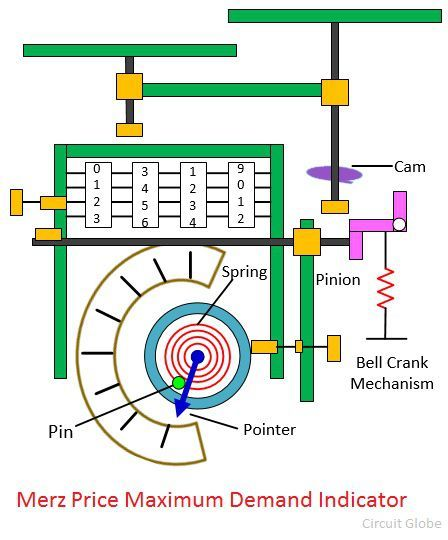# Maximum Demand Indicator

Definition: The maximum demand indicator measures the maximum amount of power requires by the consumer at the particular interval of time. The indicator is designed in such a way so that they measure the base and peak load but unable to measures the sudden short-circuit or starting high current of the motor. It is designed for recording the power over particular periods.

The maximum demand indicators are classified into four types.

1. Recording demand indicator
2. Average demand indicator
3. Thermal type maximum demand indicator
4. Digital Maximum Demand Indicator

## Construction of Maximum Demand Indicator

The maximum demand indicator has five main parts.

• Dial Connected to the moving system
• Pointer
• Reset Device
• Fraction device
• Indicating Pin

## Average Demand Indicator

The average demand indicator is inbuilt into the energy meter. The energy meter and average demand indicator together measures the total power consumes and the maximum value of specific power at particular interval of time. The average demand indicator consists the complex speed dial mechanism.The pin drive moves the dial forward for small duration (say for half an hour). The total power consumes at that interval is shown on the dial. The instrument consists the cam which is controlled by the timing gears. The cam brings back the pointer at zero positions.

The pointer records the total power consumes by the load at that particular interval of time. For the next half an hour, the pin again moved forward. But the pointer will move forward only when the total power consumed by the load is more than the previous periods.

The formula calculates the average maximum demand,The maximum demand meter can measure the power regarding kVarh or kVah. This can be done by adding the suitable meter which will calculate such quantities.

## Advantages of Average Demand Indicator

• The average demand indicator has high accuracy.
• The instrument has uniform measuring scale.

## Disadvantages of Maximum Demand Indicator

• The cost of the instrument is very high.
• Their construction is very complicated.

Nowadays, the cam is replaced by the electromagnetic relay and clutch the replaces the bell crank releasing device.